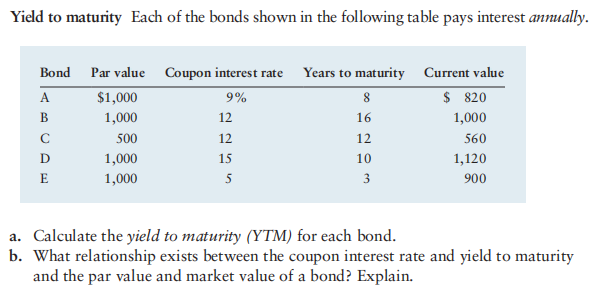# Question Yield to maturity Each of the bonds shown in the following table pays interest annually. \begin{tabular}{lcccc} Bond & Par value & Coupon interest rate & Years to maturity & Current value \\ \hline A & $$\ 1,000$$ & $$9 \%$$ & 8 & $$\ 820$$ \\ B & 1,000 & 12 & 16 & 1,000 \\ C & 500 & 12 & 12 & 560 \\ D & 1,000 & 15 & 10 & 1,120 \\ E & 1,000 & 5 & 3 & 900 \end{tabular} a. Calculate the yield to maturity (YTM) for each bond. b. What relationship exists between the coupon interest rate and yield to maturity and the par value and market value of a bond? Explain.Yield to maturity Each of the bonds shown in the following table pays interest annually.
\begin{tabular}{lcccc}
Bond & Par value & Coupon interest rate & Years to maturity & Current value \\
\hline A & $$\ 1,000$$ & $$9 \%$$ & 8 & $$\ 820$$ \\
B & 1,000 & 12 & 16 & 1,000 \\
C & 500 & 12 & 12 & 560 \\
D & 1,000 & 15 & 10 & 1,120 \\
E & 1,000 & 5 & 3 & 900
\end{tabular}
a. Calculate the yield to maturity (YTM) for each bond.
b. What relationship exists between the coupon interest rate and yield to maturity and the par value and market value of a bond? Explain.Transcribed Image Text: Yield to maturity Each of the bonds shown in the following table pays interest annually. \begin{tabular}{lcccc} Bond & Par value & Coupon interest rate & Years to maturity & Current value \\ \hline A & $$\ 1,000$$ & $$9 \%$$ & 8 & $$\ 820$$ \\ B & 1,000 & 12 & 16 & 1,000 \\ C & 500 & 12 & 12 & 560 \\ D & 1,000 & 15 & 10 & 1,120 \\ E & 1,000 & 5 & 3 & 900 \end{tabular} a. Calculate the yield to maturity (YTM) for each bond. b. What relationship exists between the coupon interest rate and yield to maturity and the par value and market value of a bond? Explain.
More
Transcribed Image Text: Yield to maturity Each of the bonds shown in the following table pays interest annually. \begin{tabular}{lcccc} Bond & Par value & Coupon interest rate & Years to maturity & Current value \\ \hline A & $$\ 1,000$$ & $$9 \%$$ & 8 & $$\ 820$$ \\ B & 1,000 & 12 & 16 & 1,000 \\ C & 500 & 12 & 12 & 560 \\ D & 1,000 & 15 & 10 & 1,120 \\ E & 1,000 & 5 & 3 & 900 \end{tabular} a. Calculate the yield to maturity (YTM) for each bond. b. What relationship exists between the coupon interest rate and yield to maturity and the par value and market value of a bond? Explain.